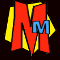#The 1089 Trick

This trick has two "magic" stages.

 Write down a three digit number, the digits must be different and the biggest digit must be at the front. Reverse the number and write it underneath. (The middle digit should stay in the middle.) Subtract the lower from the higher. (If you get "99" put a zero in front to make "099") First bit of "magic": your answer will have "9" in the middle. Also the first and last digits will add up to 9. Reverse this answer. Add the last two answers. Second bit of "magic": the total is... 1089 Example a:  741 -147 =594 +495 =1089 Example b:  938 -839 =099 +990 =1089This trick and many others are explained in the book The PHANTOM X (algebra)

### 1089 FACTS

• 332 = 1089
3332 = 110889
33332 = 11108889
333332 = 1111088889 etc.

• 1089 x 1 = 1089     Can you see the pattern?
1089 x 2 = 2178
1089 x 3 = 3267     Look down the columns of digits!
1089 x 4 = 4356
1089 x 5 = 5445
1089 x 6 = 6534
1089 x 7 = 7623
1089 x 8 = 8712
1089 x 9 = 9801 (note 9801 is 1089 reversed)

• 1/1089 = 0.00 09 18 27 36 45 54 63 72 ... (think of the nine times table!)

• 1/9801 = 0.00 01 02 03 04 05 06 07 08 09 10 11 12 13 14...